Hi.

I need to make a bar / line chart, which groups the last 12 months of the year, and shows me in bars, the sum of each of the columns.

Source of dates: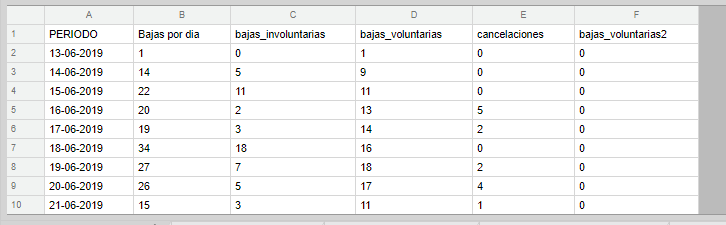Expected type of graph: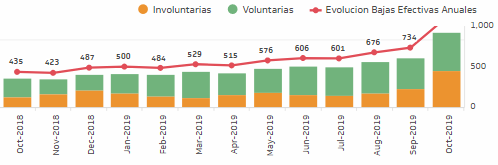X-AXIS: There are no problems here. Using the following, I manage to group by month, the last 12 months of the year: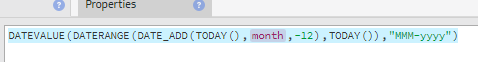SERIES:  Here comes the problem: if I do the following, I add (count) the number of rows in column A. This logically, is not what I wanted, but it gives me the idea, that the Lookup works well.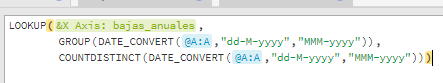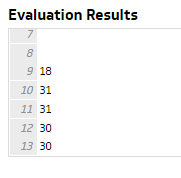If in the "countdistinct" I change column A, for column C (which would be expected) I don't get results.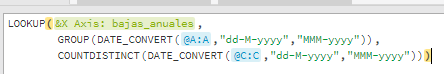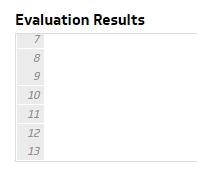I am sure that the problem is in the COUNTDISTINTC, but I could not solve it, so that I can add all the values in column C, grouped by month.

Can someone help me with this?
Thank you.

#### 1 comment

•Janice Janczyn

Hello,

When you see an issue like this, evaluate each part of your formula to find where the results are not what you expect. In this case, when you evaluate the COUNTDISTINCT( DATE_CONVERT( @C:C, "dd-M-yyyy", "MMM-yyyy" ) what results do you get? Because the data in column C is not a date, my guess is that the DATE_CONVERT returns all blanks and so the COUNTDISTINCT returns 1 value which is the count of all the blanks.

To sum the values in column C by month, use GROUPBY, by default the method applied is to sum the values in the 2nd parameter:

GROUPBY( DATE_CONVERT( @A:A, "dd-M-yyyy", "MMM-yyyy" ),
@C:C )

I hope this helps. If you have further questions, please email support@klipfolio.com.

Thanks,
Janice